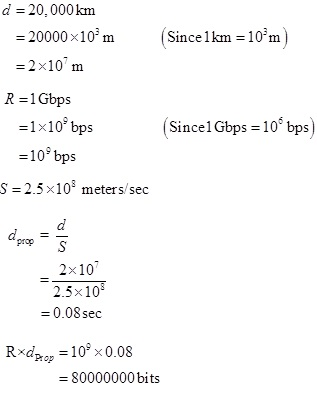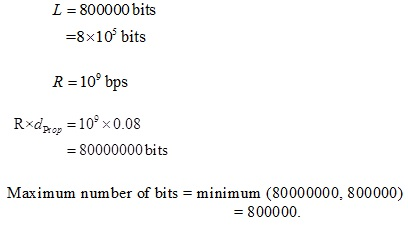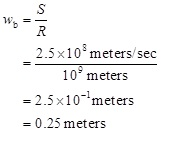SHARE
HELP

To start with, Sr2Jr’s first step is to reduce the expenses related to education. To achieve this goal Sr2Jr organized the textbook’s question and answers. Sr2Jr is community based and need your support to fill the question and answers. The question and answers posted will be available free of cost to all.Authors:
James F. Kurose, Keith W. Ross
Chapter:
Computer Networks And The Internet
Exercise:
Problems
Question:27 | ISBN:9780132856201 | Edition: 6

###Question

Consider problem P25 but now with a link of R = 1 Gbps.

a. Calculate the bandwidth-delay product, R _ dprop.

b. Consider sending a file of 800,000 bits from Host A to Host B. Suppose

the file is sent continuously as one big message. What is the maximum

number of bits that will be in the link at any given time?

c. What is the width (in meters) of a bit in the link?

###Answer

a)Therefore, the bandwidth-delay product is 8000000 bits.

b)c)Therefore, the width of a bit in the link is=0.25 meters.

### Discussions

Post the discussion to improve the above solution.